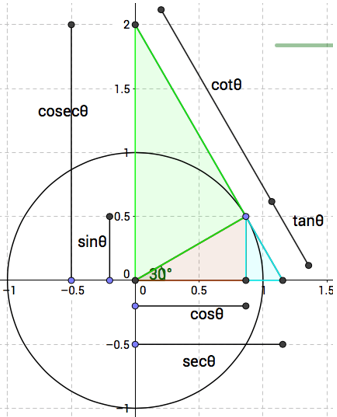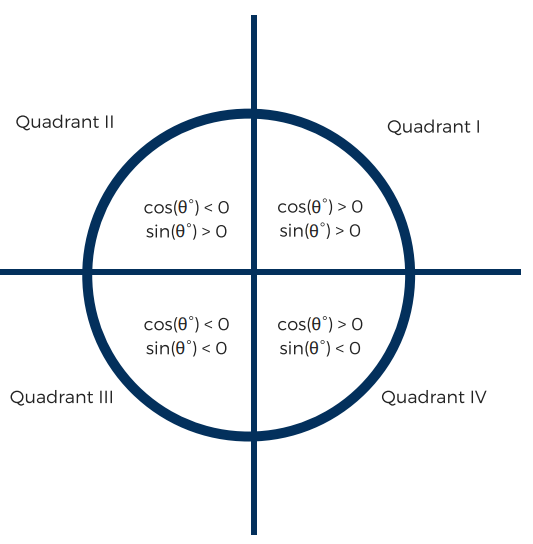# Unit Circle and Trigonometric Functions

## Objective

Define and evaluate the trigonometric functions of tangent, cosecant, secant, and cotangent.

## Common Core Standards

### Core Standards

?

• F.TF.A.2 — Explain how the unit circle in the coordinate plane enables the extension of trigonometric functions to all real numbers, interpreted as radian measures of angles traversed counterclockwise around the unit circle.

• F.TF.A.3 — Use special triangles to determine geometrically the values of sine, cosine, tangent for π/3, π/4 and π/6, and use the unit circle to express the values of sine, cosine, and tangent for π-x, π+x, and 2π-x in terms of their values for x, where x is any real number.

?

• G.SRT.C.6

## Criteria for Success

?

1. Define cosecant, secant, and cotangent in terms of sine, cosine, and tangent.
2. Evaluate all six trigonometric functions at reference angles.

## Tips for Teachers

?

• This Demos tool with the unit circle is helpful for demonstration purposes.
• "10 Secret Trig Functions your Math Teachers Never Taught You" by Evelyn Lamb on ScientificAmerican.com provides interesting context for the different trigonometric functions.

## Anchor Problems

?

### Problem 1

Below are six different trigonometric ratios, all noted on the unit circle.

${\mathrm{sec}\theta}$” is “secant of theta”
${\mathrm{cosec}\theta}$” is “cosecant of theta” (also can be written as ${\mathrm{csc}\theta}$)
${\mathrm{cot}\theta}$” is “cotangent of theta”

What is the relationship between the sine, cosine, and tangent and these three new trigonometric functions?#### Guiding Questions

Create a free account or sign in to access the Guiding Questions for this Anchor Problem.

#### References

Reciprocal Trig Functions on the Unit Circle by mathsurgery.wikispaces is made available by GeoGebra under the CC BY-NC-SA 3.0 license. Copyright © International GeoGebra Institute, 2013. Accessed Feb. 26, 2018, 1 p.m..

### Problem 2

Evaluate the following expressions:

a.    ${\mathrm{csc}(30^\circ)}$

b.    ${\mathrm{cot}(135^\circ)}$

c.    ${\frac{\mathrm{cos}(45^\circ)}{\mathrm{sec}(60^\circ)}}$

d.    ${-\mathrm{cos}(-60^\circ)}$

#### Guiding Questions

Create a free account or sign in to access the Guiding Questions for this Anchor Problem.

### Problem 3

The image below shows where the values of sine and cosine are positive and negative. Fill out a similar image for cosecant, secant, tangent, and cotangent.#### Guiding Questions

Create a free account or sign in to access the Guiding Questions for this Anchor Problem.

## Problem Set

?

The following resources include problems and activities aligned to the objective of the lesson that can be used to create your own problem set.

## Target Task

?

### Problem 1

Evaluate:

${\mathrm{tan}0^\circ}$

${\mathrm{cot}45^\circ}$

${\mathrm{csc}90^\circ}$

### Problem 2

Where is the value of ${\mathrm{sec}\theta}$ undefined?